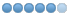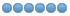# Valgrind: Memory Tool

• zusuk
•Topic Author
• Offline
• Elite Member
•• LuminariMUD Developer
10 years 8 months ago - 5 years 10 months ago #1356 by zusuk
Valgrind: Memory Tool was created by zusuk
This is taken in part from: valgrind.org/

1. Introduction

Valgrind is a memory debugging tool. It can help detect memory-related errors that are common and can lead to crashes or other unpredictable behaviour. You can check if it is installed by typing on your command prompt: valgrind --help

2. Running Valgrind

Do a clean compile on your program. Then use the command line from your MUD's directory:
valgrind --log-file="filename" --leak-check=yes bin/circle -q <port>

3. Simplified Interpreation of Output

I suggest leaving Valgrind running overnight, and using various commands - including spamming new commands you've made, etc.

Then "shutdown die" your MUD. You will see something similar to this output at the very bottom:

==7300== HEAP SUMMARY:
==7300== in use at exit: 826 bytes in 31 blocks
==7300== total heap usage: 309,395 allocs, 309,364 frees, 38,722,578 bytes allocated
==7300==
==7300== 16 (8 direct, 8 indirect) bytes in 1 blocks are definitely lost in loss record 3 of 9
==7300== at 0x4004EC2: calloc (vg_replace_malloc.c:418)
==7300== by 0x813554E: remember (mobact.c:666)
==7300== by 0x8102D51: hit (fight.c:1968)
==7300== by 0x81033ED: perform_attacks (fight.c:2130)
==7300== by 0x8103B09: perform_violence (fight.c:2422)
==7300== by 0x80C9E09: heartbeat (comm.c:1080)
==7300== by 0x80CD2C7: game_loop (comm.c:947)
==7300== by 0x80CEE86: main (comm.c:540)
==7300==
==7300== 80 (56 direct, 24 indirect) bytes in 7 blocks are definitely lost in loss record 7 of 9
==7300== at 0x4004EC2: calloc (vg_replace_malloc.c:418)
==7300== by 0x813554E: remember (mobact.c:666)
==7300== by 0x8102D51: hit (fight.c:1968)
==7300== by 0x806F913: event_whirlwind (act.offensive.c:1205)
==7300== by 0x80E0B39: event_process (dg_event.c:126)
==7300== by 0x80C9810: heartbeat (comm.c:1060)
==7300== by 0x80CD2C7: game_loop (comm.c:947)
==7300== by 0x80CEE86: main (comm.c:540)
==7300==
==7300== LEAK SUMMARY:
==7300== definitely lost: 64 bytes in 8 blocks
==7300== indirectly lost: 32 bytes in 4 blocks
==7300== possibly lost: 0 bytes in 0 blocks
==7300== still reachable: 730 bytes in 19 blocks
==7300== suppressed: 0 bytes in 0 blocks
==7300== Reachable blocks (those to which a pointer was found) are not shown.
==7300== To see them, rerun with: --leak-check=full --show-reachable=yes

Understanding Common Errors:

==12345== Invalid read/write of size X

This typically means your application is reading or writing memory from/to a location that is not allocated to it. Typical causes are trying to access memory through a pointer that has already been freed, accessing through a pointer that has never been initialized, or a buffer overflow.

==12345== Conditional jump or move depends on uninitialised value(s)

This is a typical message that you'll see when you're using the value of some variable, but the variable has never been initialized.

==12345== Invalid free()

This you'll see if you're trying to free the same memory twice, or if you're trying to free memory that wasn't allocated to you in the first place (probably trying to free an uninitialized pointer).

==12345== XX bytes in X blocks are definitely lost in loss record X of X

This is the typical message you will see when a memory leak occurs. Of course the typical way to introduce memory leaks is by allocating memory but overwriting all the pointers to it.

4. Conclusion

Valgrind isn't perfect, although its suggested that it is 99% accurate on its output.

Website
www.luminariMUD.com

Main Game Port
luminariMUD.com:4100
Last edit: 5 years 10 months ago by zusuk. Reason: Added logfile parameter in command line example

• JTP
• Offline
• Platinum Member
•10 years 4 months ago #2459 by JTP
Replied by JTP on topic Valgrind: Memory Tool

Conditional jump or move depends on uninitialised value(s)

• Vatiken
•• Offline
•• tbaMUD Programmer
10 years 4 months ago #2468 by Vatiken
Replied by Vatiken on topic Valgrind: Memory Tool
What OS?

tbaMUD developer/programmer

• WhiskyTest
•• Offline
• Platinum Member
•9 years 1 month ago #5008 by WhiskyTest
Replied by WhiskyTest on topic Valgrind: Memory Tool
I tried on stock 3.65, running on CentOS 6.4
```Aug 25 21:12:48 :: Done.
==1318==
==1318== HEAP SUMMARY:
==1318==     in use at exit: 829 bytes in 9 blocks
==1318==   total heap usage: 289,431 allocs, 289,422 frees, 113,143,305 bytes allocated
==1318==
==1318== 805 (280 direct, 525 indirect) bytes in 1 blocks are definitely lost in loss record 9 of 9
==1318==    at 0x4C2677B: calloc (vg_replace_malloc.c:593)
==1318==    by 0x448E38: do_oasis_cedit (cedit.c:78)
==1318==    by 0x49B9AF: command_interpreter (interpreter.c:582)
==1318==    by 0x450B49: game_loop (comm.c:889)
==1318==    by 0x4514C1: main (comm.c:533)
==1318==
==1318== LEAK SUMMARY:
==1318==    definitely lost: 280 bytes in 1 blocks
==1318==    indirectly lost: 525 bytes in 7 blocks
==1318==      possibly lost: 0 bytes in 0 blocks
==1318==    still reachable: 24 bytes in 1 blocks
==1318==         suppressed: 0 bytes in 0 blocks
==1318== Reachable blocks (those to which a pointer was found) are not shown.
==1318== To see them, rerun with: --leak-check=full --show-reachable=yes
==1318==
==1318== For counts of detected and suppressed errors, rerun with: -v
==1318== Use --track-origins=yes to see where uninitialised values come from
==1318== ERROR SUMMARY: 1252 errors from 40 contexts (suppressed: 6 from 6)
[root@centos6-7x64 Stock]#```

At run time here are a couple of the outputs:
```==1318==
==1318== Invalid write of size 8
==1318==    at 0x46BED1: script_driver (dg_scripts.c:2689)
==1318==    by 0x47042B: greet_mtrigger (dg_triggers.c:224)
==1318==    by 0x41F19A: do_simple_move (act.movement.c:322)
==1318==    by 0x4A3495: mobile_activity (mobact.c:89)
==1318==    by 0x44EA44: heartbeat (comm.c:988)
==1318==    by 0x4501E1: game_loop (comm.c:938)
==1318==    by 0x4514C1: main (comm.c:533)
==1318==  Address 0x7e1b960 is 80 bytes inside a block of size 104 free'd
==1318==    at 0x4C273F0: free (vg_replace_malloc.c:446)
==1318==    by 0x45EA28: extract_script (dg_handler.c:157)
==1318==    by 0x469493: process_detach (dg_scripts.c:1896)
==1318==    by 0x46C9CE: script_driver (dg_scripts.c:2657)
==1318==    by 0x47042B: greet_mtrigger (dg_triggers.c:224)
==1318==    by 0x41F19A: do_simple_move (act.movement.c:322)
==1318==    by 0x4A3495: mobile_activity (mobact.c:89)
==1318==    by 0x44EA44: heartbeat (comm.c:988)
==1318==    by 0x4501E1: game_loop (comm.c:938)
==1318==    by 0x4514C1: main (comm.c:533)
==1318==
==1318== Invalid read of size 8
==1318==    at 0x470350: greet_mtrigger (dg_triggers.c:215)
==1318==    by 0x41F19A: do_simple_move (act.movement.c:322)
==1318==    by 0x4A3495: mobile_activity (mobact.c:89)
==1318==    by 0x44EA44: heartbeat (comm.c:988)
==1318==    by 0x4501E1: game_loop (comm.c:938)
==1318==    by 0x4514C1: main (comm.c:533)
==1318==  Address 0x7e1b968 is 88 bytes inside a block of size 104 free'd
==1318==    at 0x4C273F0: free (vg_replace_malloc.c:446)
==1318==    by 0x45EA28: extract_script (dg_handler.c:157)
==1318==    by 0x469493: process_detach (dg_scripts.c:1896)
==1318==    by 0x46C9CE: script_driver (dg_scripts.c:2657)
==1318==    by 0x47042B: greet_mtrigger (dg_triggers.c:224)
==1318==    by 0x41F19A: do_simple_move (act.movement.c:322)
==1318==    by 0x4A3495: mobile_activity (mobact.c:89)
==1318==    by 0x44EA44: heartbeat (comm.c:988)
==1318==    by 0x4501E1: game_loop (comm.c:938)
==1318==    by 0x4514C1: main (comm.c:533)
==1318==
==1318== Source and destination overlap in strcpy(0x7fefff660, 0x7fefff662)
==1318==    at 0x4C29038: strcpy (mc_replace_strmem.c:442)
==1318==    by 0x485F3B: get_number (handler.c:602)
==1318==    by 0x487FB2: get_obj_in_list_vis (handler.c:1150)
==1318==    by 0x41A65D: do_drop (act.item.c:590)
==1318==    by 0x49B9AF: command_interpreter (interpreter.c:582)
==1318==    by 0x450B49: game_loop (comm.c:889)
==1318==    by 0x4514C1: main (comm.c:533)```

Is this normal/expected output?

Another question, what is the OS that is hosting tbaMUD? I'm wondering if CentOS is doing something a little unexpected and would like to try in on a different flavor of Linux.

• thomas
•• Offline
•